## Find how many solutions there are to the given equation that satisfy the given condition. y1 +y2+y3+y4=27, each yi is a nonnegative in

Question

Find how many solutions there are to the given equation that satisfy the given condition.
y1 +y2+y3+y4=27, each yi is a nonnegative integer.

in progress 0
5 months 2021-08-23T10:40:52+00:00 1 Answers 12 views 0

17550 solutions

Step-by-step explanation:

Given that:

y1 +y2+y3+y4=27

where;

(yi  ≥ 0 and yi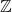)

The no. of a nonnegative integer determines the number of ways to choose 27 objects from (4) distinct objects with repetition regardless of the order.

i.e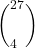The number of nonnegative integer solution is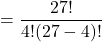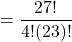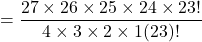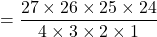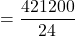= 17550 solutions